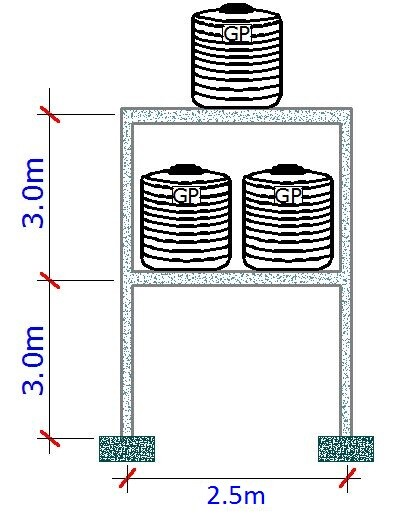# Free Vibration of Water Tank Stand When Filled With WaterIt is desired to find the eigenfrequencies and eigenvectors (modal parameters) of concrete water tank support when filled with water. This is a case of undamped free vibration, treating the stored water as a lumped mass on the tank stand. The tank will be treated as a system with 2 degrees of freedom, considering lateral displacement only.

Density of concrete = 24 KN/m3
Thickness of slab = 150mm
Dimension of columns = 230 x 230mm
Supporting beams = 300 x 230mm
Weight of water = 10 KN/m3
Size of tank = 2000 litres
Modulus of elasticity of concrete = 21.7 × 106 KN/m2

Self weight of slab = 24 KN/m3 × 0.15m × 2.5m × 2.5m = 22.5 KN = 2293.75 kg
Weight of supporting beams on the four sides = 4 × 24 KN/m3 × 2.27m × 0.23m × 0.3m = 15.036 KN = 1532.77kg
Weight of water = 10 KN/m3 × 2m3 = 20 KN = 2038.73 kg
Weight of tank (assume) = 75kg

At the 1st level = 2293.75 + 1532.77 + 2038.73 + 75 = 5940.25 kg
At the 2nd level = 2293.75 + 1532.77 + 2(2038.73) + 2(75) = 8053.98 kg

We are assuming that the floor slab is very stiff compared to the columns. So the flexural rigidity is taken as infinity.

Geometrical properties of the sections
Columns = 23cm x 23cm = 0.23m x 0.23m
Moment of inertia of column IC = (0.23 × 0.233)/12 = 2.332 × 10-4 m4
EIC = (2.332 × 10-4 m4) × (2.1 × 107 KN/m2) = 4897.2 KN.m2

The mass matrix of the structure is given below;

Determination of the stiffness matrix

(12EIC)/L3 = (12 × 4897.2)/33 = 2176.533 KN/m
K11 = (12EIC)/h3 + (12EIC)/h3
K11 = 2176.533 + 2176.533 = 4353.067
K21 = -(2176.533 + 2176.533) = -4353.067
K22 = 2(2176.533) + 2(2176.533) = 8706.132

The stiffness matrix is therefore;

For an undamped free vibration, the equation of motion is;

Mü + ku = 0 —————– (1)

Arranging this in matrix form, we obtain;

The characteristic polynomial equation is given by;

On solving;

((4353.067 × 103 – 5940.25ω2) × (8706.132 × 103 – 8053.98ω2) – (4353.067 × 103 × 4353.067 × 103) = 0

On simplifying;

(4.783 × 1074 – (8.677 × 10102 + (1.8949 × 1013) = 0

On solving;

The natural frequencies;
F1 = ω1/2π = 2.5361 Hz
F2 = ω2/2π = 6.2858 Hz

Determination of the mode shapes

Considering the first row of the matrix;
(2.8448 × 106)A1 – (4353.067 × 103)A2 = 0

Setting A1 = 1.0
A2 = (2.8448 × 106)/(4353.067 × 103) = 0.6780
Therefore;ϕ1 = [1, 0.6535]T

Considering the first row of the matrix;
(-4.9129 × 106)A1 – (4353.067 × 103)A2 = 0

Setting A1 = 1.0
A2 = (-4.9129 × 106)/(4353.067 × 103) = -1.128
Therefore;ϕ2 = [1, -1.128]T

The generalised mass and stiffness of the structure (modal mass and stiffness);

As a verification;
K1/M1 = 2381668.751 / 9379.8 = ω12 = 253.9146 rad2/sec2
K2/M2 = 25251112,921 / 9379.8 = ω22 = 1559.9 rad2/sec2

Eigenvector Mass Orthonormalization
The idea is to obtain;

ϕ1T1 = 1.0

The conversion factor;

Therefore, C1 = 1/√(9379.8) = 0.0103

Hence;

Similarly;

Therefore, C2 = 1/√(16188.005) = 0.00785

Hence;

We can therefore obtain;

Let χi(t) be the generalised coordinate which represent the amplitude of the orthonormalised mode shape.

The displacement time history response is therefore; u(t) = Φχ(t)

But the modal equation of motion

Mü + ku = 0 —————– (1)

Realise that;

Substituting ü and u into equation 1 and multiplying by ΦT , we obtain;

ΦT MΦẍ + ΦT KΦx = 0

ẍ + Kgx = 0

This leads to the following differential equations;

1(t) + 253.9146x1(t)  = 0 ——————– (a)
2(t) + 1559.9x2(t)  = 0 ——————– (b)

Let the initial condition be a unit velocity;
x1(0) = 0;  ẋ1(0)  = 0

Solving the differential equation by Laplace Transform;

For mode 1;
1(t) + 253.9146x1(t)  = 0    x1(0) = 0; ẋ1(0)  = 1.0

(s2x ̅  – sx0 – x1) + 253.9146x ̅ = 0
s2x ̅  – 1 + 253.9146x ̅ = 0
x ̅ (s2   + 253.9146) = 1
x ̅ = 1 / (s2   + 253.9146)

On solving by Laplace Transform;

x1 = (1/√253.9146) sin(√253.9146)
x1 = 0.0627 sin(15.935t)

For mode 2;
2(t) + 1559.96x2(t)  = 0    x1(0) = 0; ẋ1(0)  = 1.0

(s2x ̅  – sx0 – x1) + 1559.9x ̅ = 0
s2x ̅  – 1 + 1559.9x ̅ = 0
x ̅ (s2   + 1559.9) = 1
x ̅ = 1 / (s2   + 1559.9)

On solving by Laplace Transform;

x2 = (1/√1559.9) sin(√1559.9)
x2 = 0.0253 sin(39.495t)

Knowing that
u(t) = Φχ(t)

The displacement time history is therefore;

u1(t) = 0.00064581 sin(15.935t) + 0.0001986 sin(39.495t)
u2(t) = 0.0004219 sin(15.935t) – 0.000224 sin(39.495t)

The displacement time history graph is shown below;

Thank you for visiting Structville. God bless.

1. Hello Bro,

I think there are a few typo's in your post, specifically Load Analysis.

What would you consider to be your effective span of slab and beam?

Beam DIm (230mm x 300mm)

Wouldn't your effective span be 3 metres ?

Fc

2. Thank you for the observation. I used a former picture and the correction has been effected.

3. This may be a trivial question, I am assuming that the tanks are some how connected to the slabs hence the inclusion of the mass?

what would be the dynamic effect if only in contact with slab due to gravity and you introducing a vibration?

Fc

6.UK Vapor Waves